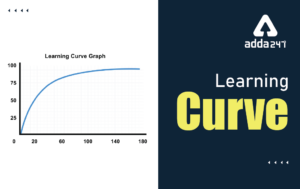# Normal Probability Distribution : Download Study Notes for All Teaching Exam

DSSSB PRT Study Notes: Delhi Subordinate Staff Selection Board (DSSSB) is going to conduct the most prestigious exam in the field of government teaching jobs this year 2021. As DSSSB released more than 12000 teaching vacancies i.e. TGT, PRT post, students had already started preparing hard for this exam. Teaching Aptitude & methodology is a big topic for the DSSSB PRT exam which contains 100 questions for 100 marks. This topic contains teaching strategy, teaching methodology, learning principles, application-based questions and classroom management, etc. Here we are going to learn with Teaching Study notes for better preparation.

Are You Preparing For DSSSB Exam? Grab This Opportunity NowENROLL NOW

FREE STUDY Material FOR DSSSB TGT PRT Exam

## Normal Probability Distribution

Definition : Probability Density Function

A probability density function is an equation used to compute probabilities of continuous random variables that must satisfy the following two properties.

1. Graph of the equation must be greater than or equal to zero for all possible values of the random variable.
2. Area under the curve equals 1.

EXAMPLE : Illustrating the Uniform Distribution

Imagine that a friend of yours is always late. Let the random variable X represent the time from when you are supposed to meet your friend until he shows up. Further suppose that your friend could be on time (x = 0) or up to 30 minutes late (x = 30) with all 1 – minute intervals of times between x = 0 and x = 30 equally likely. That is to say, your friend is just as likely to be from 3 to 4 minutes late as he is to be 25 to 26 minutes late. The random variable X can be any value in the interval from 0 to 30, that is, 0 ≤  X ≤  30. Because any two intervals of equal length between 0 and 30, inclusive, are equally likely, the random variable X is said to follow a uniform probability distribution.

Properties of the Normal Probability Curve :

1. The highest point occurs at x = µ.
2. It is symmetric about the mean, µ. One half of the curve is a mirror image of the other half, i.e., the area under the curve to the right of µ is equal to the area under the curve to the left of µ equals ½.
3. It has inflection points at µ – σ and µ + σ.
4. The curve is asymptotic to the horizontal axis at the extremes.
5. The total area under the curve equals one.Properties of the Normal Probability Curve (continued) :

1. Empirical Rule :
• Approximately 68 % of the area under the curve is between µ – σ and µ + σ.
• Approximately 95 % of the area under the curve is between µ – 2σ and µ + 2σ.
• Approximately 99.7 % of the area under the curve is between µ – 3σ and µ + 3σ.A normal curve has two characteristics : mean (µ) and standard deviation (σ).

Example 1 – normal curves for two populations with different means :Summary : The two curves are exactly the same, except one curve is to the right of the other curve.

Example 2 – normal curves for two populations with different standard deviations.Practice DSSSB Exam with Study Plan

Summary : Increasing the standard deviation causes the curve for Population #2 to become flatter and more spread out. Comparing the two normal curves :

• For Population #1, there is more area under the curve within a given distance of the mean;
• For Population #2, there is more area under the curve away from the mean.

Standardized Variable – A variable is said to be standardized if it has been adjusted (or transformed) such that its mean equals 0 and its standard deviation equals 1.The z – score represents the number of standard deviations that a data value is away from the mean.

Normal Probability Distributions (or Curves).

• A normal curve is characterized by its mean, µ, and standard deviation, σ.
• Since there are an infinite number of combinations of µ’s and σ’s, there are likewise an infinite number of normal curves.
• One particular type of normal curve is the standard normal curve…a normal curve with µ = 0 and σ = 1.

The Standard Normal Distribution

Standardizing a Normal Random Variable

Suppose the random variable X is normally distributed with mean µ and standard deviation σ. Then the random variableis normally distributed with mean µ = 0 and standard deviation σ = 1. The random variable Z is said to have the standard normal distribution.

Standard Normal Distribution (Z)Properties of the Standard Normal Curve (Z) :

1. The highest point occurs at µ = 0.
2. It is a bell – shaped curve that is symmetric about the mean, µ = 0. One half of the curve is a mirror image of the other half, i.e., the area under the curve to the right of µ = 0 is equal to the area under the curve to the left of µ = 0 equals ½.
3. It has inflection points at µ – σ = 0 – 1 = – 1 and µ + σ = 0 + 1 = + 1.
4. The curve is asymptotic to the horizontal axis at the extremes.
5. The total area under the curve equals one.
6. Empirical Rule :
• Approximately 68 % of the area under the curve is between – 1 and + 1.
• Approximately 95 % of the area under the curve is between – 2 and + 2.
• Approximately 99.7 % of the area under the curve is between – 3 and + 3.

•Learning Curve - What is Learning Curve?...
•रस - परिभाषा, भे�...
•पठन कौशल- परिभा�...
•अलंकार - परिभाष�...
•Indian States and Capitals 2022: 28 Stat...
•New Education Policy(NEP) PDF - नई �...